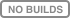# bevelOrdinal regression refers to a number of techniques that are designed to classify inputs into ordered (or ordinal) categories. This type of data is common in social science research settings where the dependent variable often comes from opinion polls or evaluations. For example, ordinal regression can be used to predict the letter grades of students based on the time they spend studying, or Likert scale responses to a survey based on the annual income of the respondent.

In People Analytics at Shopify, we use ordinal regression to empower Shopify employees. Our annual engagement survey contains dozens of scale questions about wellness, team health, leadership and alignment. To better dig into this data we built `bevel`, a repository that contains simple, easy-to-use Python implementations of standard ordinal regression techniques.

### Using bevel

#### Fitting

The API to bevel is very similar to scikit-learn's API. A class is instantiated that has a `fit` method that accepts the design matrix (also called the independent variables) and the outcome array (also called the dependent variable). For bevel, the outcome array contains values from a totally-orderable set (example: {0, 1, 2, ...}, {'A', 'B', 'C', ...}, {'01', '02', '03', ...}) representing your ordinal data. (This may require some pre-processing map, for example, encoding survey responses into integers.)

The design matrix can be a numpy array, or a pandas DataFrame. The benefit of using the latter is that the DataFrame column names are displayed in inference later.

Below is an example of fitting with the `OrderedLogit` model.

```from bevel.linear_ordinal_regression import OrderedLogit

ol = OrderedLogit()
ol.fit(X, y)
```
##### Inference and prediction

After bevel fits the model to the data, additional methods are available to use. To see the coefficients of the fitted linear model, including their standard errors and confidence intervals, use the `print_summary` method. Below is the output of the UCLA dataset.

```ol.print_summary()
"""
beta  se(beta)      p  lower 0.95  upper 0.95
attribute names
pared            1.0477    0.2658 0.0001      0.5267      1.5686  ***
public          -0.0587    0.2979 0.8439     -0.6425      0.5251
gpa              0.6157    0.2606 0.0182      0.1049      1.1266    *
---
Signif. codes:  0 '***' 0.001 '**' 0.01 '*' 0.05 '.' 0.1 ' ' 1

Somers' D = 0.158
"""
```

These values as a pandas DataFrame are available on the `summary` property of the fitted class. The `Somers' D` value is a measure of the goodness-of-fit of the model, analogous to the R² value in ordinary linear regression. However, unlike R², it can vary between -1 (totally discordant) and 1 (totally concordant).

Another goal of fitting is predicting outcomes from new datasets. For this, bevel has three prediction methods, depending on your goal.

```ol.predict_probabilities(X)  # returns a array with the probabilities of being in each class.
ol.predict_class(X)  # returns the class with the highest probability
ol.predict_linear_product(X)  # returns the dot product of X and the fitted coefficients
```

Get A Weekly Email With Trending Projects For These Topics
No Spam. Unsubscribe easily at any time.
python (53,198
inference (97
prediction (68
pandas-dataframe (32